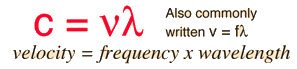# The Electromagnetic Spectrum

Click on any part of the spectrum for further detail.Speed of light### Wavelength

 λ= x 10^m

= m
= nm
= microns

### Frequency

 ν=f = x 10^Hz

= kHz = MHz
= GHz
= 1/cm (wavenumber)
Quantum energy =hν = hf = x 10^ eV

### Example of scale with U.S. common units

Index

Visualization in "Practically Science"

 HyperPhysics***** Quantum Physics R Nave
Go Back

# The Electromagnetic Spectrum### Calculation

Index

 HyperPhysics***** Quantum Physics R Nave
Go Back